How to use auto encoder for unsupervised learning models?
MACHINE LEARNING RECIPES DATA CLEANING PYTHON DATA MUNGING PANDAS CHEATSHEET     ALL TAGS

# How to use auto encoder for unsupervised learning models?

This Pytorch recipe trains an autoencoder neural net by compressing the MNIST handwritten digits dataset to only 3 features.

0

This recipe builds an autoencoder for compressing the number of features in the MNIST handwritten digits dataset.

For building an autoencoder, three components are used in this recipe :
- an encoding function,
- a decoding function,
- a loss function between the amount of information loss between the compressed representation of your data and the decompressed representation.

What is an Auto encoder ?
An auto encoder is used to encode features so that it takes up much less storage space but effectively represents the same data. It is a type of neural network that learns efficient data codings in an unsupervised way. The aim of an autoencoder is to learn a representation for a dataset, for dimensionality reduction, by ignoring signal "noise".

What is Unsupervised learning model ?
Unsupervised machine learning models infer patterns from a dataset without reference to known, or labeled, outcomes. Unlike supervised machine learning, unsupervised machine learning methods cannot be directly applied to a problem because you have no idea what the values for the output data might be, making it impossible for you to train the algorithm the way you normally would. Unsupervised learning can instead be used for discovering the underlying structure of the data.

In :
```import torch
import torch.nn as nn
import torch.utils.data as Data
import torchvision
import matplotlib.pyplot as plt
from mpl_toolkits.mplot3d import Axes3D
from matplotlib import cm
import numpy as np
%matplotlib inline
```
In :
```torch.manual_seed(1)    # reproducible
```
Out:
`<torch._C.Generator at 0x12214f310>`
In :
```# Hyper Parameters
EPOCH = 10
BATCH_SIZE = 64
LR = 0.005         # learning rate
N_TEST_IMG = 5
```
In :
```# Mnist digits dataset
train_data = torchvision.datasets.MNIST(
root='./mnist/',
train=True,
# this is training data
transform=torchvision.transforms.ToTensor(),
# Converts a PIL.Image or numpy.ndarray to

# torch.FloatTensor of shape (C x H x W) and normalize in the range [0.0, 1.0]
)
```
In :
```# plot one example
print(train_data.train_data.size())     # (60000, 28, 28)
print(train_data.train_labels.size())   # (60000)
plt.imshow(train_data.train_data.numpy(), cmap='gray')
plt.title('%i' % train_data.train_labels)
plt.show()
```
```torch.Size([60000, 28, 28])
torch.Size()
```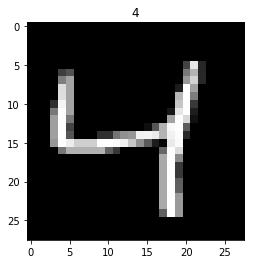In :
```# Data Loader for easy mini-batch return in training, the image batch shape will be (50, 1, 28, 28)
```
In :
```class AutoEncoder(nn.Module):
def __init__(self):
super(AutoEncoder, self).__init__()

self.encoder = nn.Sequential(
nn.Linear(28*28, 128),
nn.Tanh(),
nn.Linear(128, 64),
nn.Tanh(),
nn.Linear(64, 12),
nn.Tanh(),
nn.Linear(12, 3),   # compress to 3 features which can be visualized in plt
)
self.decoder = nn.Sequential(
nn.Linear(3, 12),
nn.Tanh(),
nn.Linear(12, 64),
nn.Tanh(),
nn.Linear(64, 128),
nn.Tanh(),
nn.Linear(128, 28*28),
nn.Sigmoid(),       # compress to a range (0, 1)
)

def forward(self, x):
encoded = self.encoder(x)
decoded = self.decoder(encoded)
return encoded, decoded
```
In :
```autoencoder = AutoEncoder()
print(autoencoder)

loss_func = nn.MSELoss()

# original data (first row) for viewing
view_data = Variable(train_data.train_data[:N_TEST_IMG].view(-1, 28*28).type(torch.FloatTensor)/255.)
```
```AutoEncoder(
(encoder): Sequential(
(0): Linear(in_features=784, out_features=128, bias=True)
(1): Tanh()
(2): Linear(in_features=128, out_features=64, bias=True)
(3): Tanh()
(4): Linear(in_features=64, out_features=12, bias=True)
(5): Tanh()
(6): Linear(in_features=12, out_features=3, bias=True)
)
(decoder): Sequential(
(0): Linear(in_features=3, out_features=12, bias=True)
(1): Tanh()
(2): Linear(in_features=12, out_features=64, bias=True)
(3): Tanh()
(4): Linear(in_features=64, out_features=128, bias=True)
(5): Tanh()
(6): Linear(in_features=128, out_features=784, bias=True)
(7): Sigmoid()
)
)
```
In :
```for epoch in range(EPOCH):
for step, (x, y) in enumerate(train_loader):
b_x = Variable(x.view(-1, 28*28))   # batch x, shape (batch, 28*28)
b_y = Variable(x.view(-1, 28*28))   # batch y, shape (batch, 28*28)
b_label = Variable(y)               # batch label

encoded, decoded = autoencoder(b_x)

loss = loss_func(decoded, b_y)      # mean square error

if step % 500 == 0 and epoch in [0, 5, EPOCH-1]:
print('Epoch: ', epoch, '| train loss: %.4f' % loss.data)

# plotting decoded image (second row)
_, decoded_data = autoencoder(view_data)

# initialize figure
f, a = plt.subplots(2, N_TEST_IMG, figsize=(5, 2))

for i in range(N_TEST_IMG):
a[i].imshow(np.reshape(view_data.data.numpy()[i],
(28, 28)), cmap='gray');
a[i].set_xticks(()); a[i].set_yticks(())

for i in range(N_TEST_IMG):
a[i].clear()
a[i].imshow(np.reshape(decoded_data.data.numpy()[i],
(28, 28)), cmap='gray')
a[i].set_xticks(()); a[i].set_yticks(())
plt.show(); #plt.pause(0.05)
```
```Epoch:  0 | train loss: 0.2333
```
```/Applications/anaconda3/lib/python3.6/site-packages/ipykernel_launcher.py:15: UserWarning: invalid index of a 0-dim tensor. This will be an error in PyTorch 0.5. Use tensor.item() to convert a 0-dim tensor to a Python number
from ipykernel import kernelapp as app
```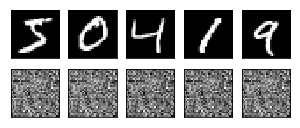```Epoch:  0 | train loss: 0.0612
```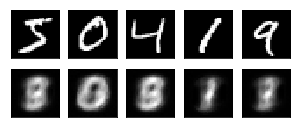```Epoch:  5 | train loss: 0.0383
```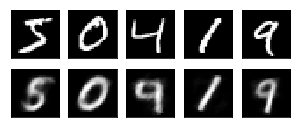```Epoch:  5 | train loss: 0.0399
```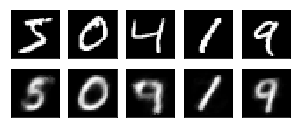```Epoch:  9 | train loss: 0.0387
```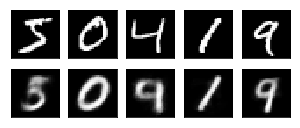```Epoch:  9 | train loss: 0.0382
```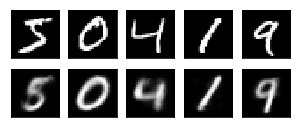#### Relevant Projects

##### Machine Learning project for Retail Price Optimization
In this machine learning pricing project, we implement a retail price optimization algorithm using regression trees. This is one of the first steps to building a dynamic pricing model.

##### Resume parsing with Machine learning - NLP with Python OCR and Spacy
In this machine learning resume parser example we use the popular Spacy NLP python library for OCR and text classification.

##### Loan Eligibility Prediction using Gradient Boosting Classifier
This data science in python project predicts if a loan should be given to an applicant or not. We predict if the customer is eligible for loan based on several factors like credit score and past history.

##### Sequence Classification with LSTM RNN in Python with Keras
In this project, we are going to work on Sequence to Sequence Prediction using IMDB Movie Review Dataset​ using Keras in Python.

##### Forecast Inventory demand using historical sales data in R
In this machine learning project, you will develop a machine learning model to accurately forecast inventory demand based on historical sales data.

##### Perform Time series modelling using Facebook Prophet
In this project, we are going to talk about Time Series Forecasting to predict the electricity requirement for a particular house using Prophet.

##### Time Series Forecasting with LSTM Neural Network Python
Deep Learning Project- Learn to apply deep learning paradigm to forecast univariate time series data.

##### Data Science Project in Python on BigMart Sales Prediction
The goal of this data science project is to build a predictive model and find out the sales of each product at a given Big Mart store.

##### Data Science Project on Wine Quality Prediction in R
In this R data science project, we will explore wine dataset to assess red wine quality. The objective of this data science project is to explore which chemical properties will influence the quality of red wines.

##### Natural language processing Chatbot application using NLTK for text classification
In this NLP AI application, we build the core conversational engine for a chatbot. We use the popular NLTK text classification library to achieve this.